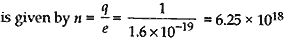Monday , May 23 2022# Electricity: 10th CBSE Science Physics

#### Question: (a) State the commercial unit of electric energy and find its relation with its SI unit. (b) The current through a resistor is made three times its initial value. Calculate how it will affect the heat produced in the resistor. (c) Find the increase in the amount of heat generated in a conductor if another conductor of double resistance is connected in the circuit keeping all other factors unchanged.

Answer: (a) The commercial unit of electric energy is kilowatt hour (kWh).
SI unit of electrical energy is Joule (J)
1 kWh = 1000 watts × 60 min
= 1000 × 60 × 60 s
= 3.6 × 106 watts seconds
= 3.6 × 106  Joules

(b) Initial production of heat
H = I²Rt
With the new resistance, heat produced
= (3I)² × R × t
= 9 I²Rt
So the heat produced will be nine times.

(c) Initial heat, H = I² × R × t
New resistance = R + 2R = 3R
With the new resistance, heat produced
= I² × 3R × t
= 3I²Rt
∴ The heat produced will become thrice.

#### Question: State Ohm’s law. How can it be verified?

Answer: It states “Physical conditions’ remaining same, the current flowing through a conductor is directly proportional to the potential difference across its two ends”.
i.e.,  V ∝ I
or V = IR,
where the constant of proportionality R is called the electrical resistance or resistance of the conductor.
Verification: The following circuit diagram is used to verify Ohm’s law.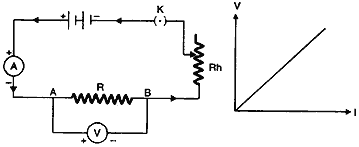Take a few cells; connect one cell across a nichrome wire AB, along with an ammeter and a voltmeter as shown in figure. Note the voltage and the current from the voltmeter and the ammeter. Now, connect two cells and again note the voltage and the current. Repeat the procedure for three cells and four cells. Calculate the ratio for each set. You will find the ratio is the nearly same in all the cases. If a graph of current against voltage in plotted, it will turn to be a straight line as shown in figure. This shows that the current is directly proportional to the potential difference.

#### (c) An electric circuit consisting of a 0.5 m long nichrome wire XY, an ammeter, a voltmeter, four cells of 1.5 V each and a plug key was set up. (i) Draw a diagram of this electric circuit to study the relation between the potential difference maintained electric current flowing through XY. (ii) Following graph was plotted between V and I values: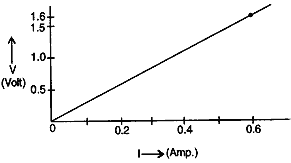#### What would be the values of V/I ratios when the potential difference is 0.8 V, 1.2 V and 1.6 V respectively? What conclusion do you draw from these values?

Answer: (a) An ammeter measures electric current in an electric circuit.
If one coulomb of charge flows through a circuit in one second, then the current in the circuit is said to be one ampere.
(b) (i) Variable resistor and (ii) closed key.
(c) (i) The circuit diagram is as shown below: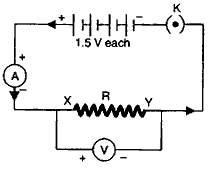(ii) Since the graph is a straight line, therefore, the value of V/I at every point will be same. This value gives the resistance of the circuit for which these V and I values are obtained. For a potential of 1.6 A, therefore, resistance of the circuit is
R = V/I = 1.6/0.6 = 2.67 Ω

#### Question: (a) (i) What is meant by saying that the potential different between two points is 1 volt? (ii) How much energy is given to 5 coulomb of charge passing through a 12 V battery? (b) Describe an activity with necessary electric circuit drawn to study the factors on which the resistance of a conducting wire depends.

(i) It means that the work done in moving a charge of a coulomb between the two points is 1 joule.
(ii) Using the expression
W = qV = 5 × 12 = 60 J

(b) Complete an electric circuit consisting of a cell, an ammeter, a nichrome wire of length l [say, marked (i)] and a plug key, as shown in Fig.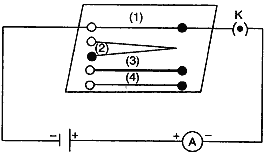1. Now, plug the key. Note the current in the ammeter.
2. Replace the nichrome wire by another nichrome wire of same thickness but twice the length, that is 2l [marked (2) in the Fig.].
4. Now, replace the wire by a thicker nichrome wire, of the same length l [marked (3)]. A thicker wire has a larger cross-sectional area. Again, note down the current through the circuit.
5. Instead of taking a nichrome wire, connect a copper wire [marked (4) in fig.] in the circuit. Let the wire be of the same length and same area of cross-section as that of the first nichrome wire [marked (1)]. Note the value of the current.
6. Notice the difference in the current in all cases.

One will notice that the current in all the three cases in different. This shows that the resistance depends upon a number of factors.

#### (b) Draw a schematic diagram of a circuit consisting of a battery of 12 V, three resistors of  5 Ω, 10 Ω and 20 Ω connected in parallel, an ammeter to measure the total current through the circuit, a voltmeter to measure the potential difference across the combination of resistors. (c) State any one advantage of connecting electrical devices in parallel with the mains instead of connecting them in series in a household circuit.

(i) A variable resistor or rheostat. Used to very current in a circuit.
(ii) Wires crossing without joining. Used when different parts of the circuit are to be connected without joining all of them.
(b) The circuit diagram is as shown below: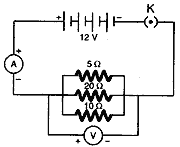(c)
(i) When the devices are connected in parallel, then even if one device if one device fails, the others keep on working.
(ii) In parallel, the potential across each device is same.

#### Question: (1). Why is the series arrangement not used for domestic circuits? (2). Why is the tungsten used almost exclusively for filament of electric lamps? (3). Why are the conductors of electric heating devices such as bread toasters and electric irons made of an alloy rather than a pure metal? (4). Why are copper and aluminium wires usually employed for electricity transmission? (5). Why does the cord of an electric heater not glow while the heating element does?

1. In the series arrangement, it any of the components stops working, then the circuit gets broken up and none of the device will work.
2. High resistivity and high melting point.
3. Alloys have a higher value of resistivity and produce more heat. They do not oxidize at high temperatures.
4. They have very low values of resistivity and current flows easily.
5. Cord of an electric heater has very low resistance, heating element is made-up of a material having high resistance.

#### (b) How many 176 Ω resistors in parallel are required to carry 5 A on a 220 V line? (c) Define electric power. Derive relation between power, potential difference and resistance.

The resistance is given by R = V/I
= 2/0.1 = 20 Ω
(b)
Resistance of the circuit with 176 Ω resistors inn parallel R = V/I
= 220/5 = 44 Ω
Let n resistors of 176 Ω be connected in parallel, then
1/44 = n/176
Hence, n = 176/44 = 4
(c)
The rate at which electric energy is dissipated or consumed in an electric circuit is called electric power.
We know that, P = W/t. Now, W = V²t/R, therefore,
P = W/t = V²t/Rt = V²/R

#### Question: (a) Three resistors R1, R2  and R3  are connected in parallel and the combination is connected to battery, ammeter, voltmeter and key, Draw suitable circuit diagram. Obtain an expression for the effective resistance of the combination of resistors in parallel. (b) Why are electric bulbs filled with chemically inactive nitrogen or argon? (c) What is meant by the statement that the rating of a fuse in a circuit is 5 A?

Answer: (a) The diagram is as shown: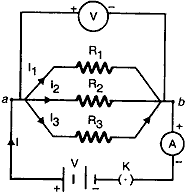(a) See above articles.
(b) They are filled with inactive nitrogen or argon to prolong the life of the filament.
(c) It means that the maximum current that should flow in the circuit is 5 A. If it exceeds this value, the fuse will blow off.

#### Question: (a) In the circuit shown connect a nichrome wire of length “L” between points X and Y and note the ammeter reading. (i) When this experiment is repeated by inserting another nichrome wire of the same thickness but twice length (2L), what changes are observed in the ammeter reading? (ii) State the changes that are observed in the ammeter reading if we double the area of cross-section without changing the length in the above experiment. Justify your answer in both the cases. (b) “Potential different between points A and B in an electric field is 1 V”. Explain the statement.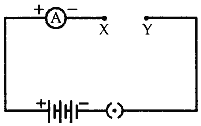(i) The ammeter reading will decrease (become half). This is because with increase in length, resistance of the circuit increases hence, current decreases.
(ii) The ammeter reading will increase (become two times). This is because as area increases, resistances and hence, current increases.

(b) It means that 1 J of work is being done to move a charge of 1 coulomb from point A to point B.

#### Question: (a) What is an electric circuit? (b) Calculate the number of electron that flow per second to constitute a current of one ampere. Charge on the electron is 1.6 × 10-19 C. (c) Draw an electric circuit of studying Ohm’s law. Label the circuit component used to measure electric current and potential difference.

It is a continuous and closed path in which an electric current can flow.

(b)
We know that I = Q/t and Q = ne, where n is the number of electron and e is the charge on the electron. Therefore, we have
I = ne/it
or  n = I t/q = 1 × 1 /1.6 × 10-19
= 6.25 × 1018 electrons.

(c) The circuit diagram used to study Ohm’s law is as shown: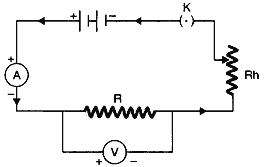Electric current: Ammeter (A).
Potential difference: Voltmeter (V).

#### Question: What does an electric circuit mean?

Answer: Electric circuit is a continuous and closed path made of conducting wires, through which the electric current flows. It comprises a cell, ammeter, voltmeter, plug key, etc.

#### Question:Define the unit of current.

Answer: SI unit of electric current is ampere (A).
Ampere is the flow of electric charges through an area at the rate of one coulomb per second, i.e. if 1 coulomb of electric charge flows through a cross-section of wire for 1 second, then it would be equal to 1 ampere.

#### Question: Calculate the number of elements constituting one coulomb of charge.

Answer: The charge on an electron is 1.6 × 10C. Therefore, the number of electrons in one coulomb